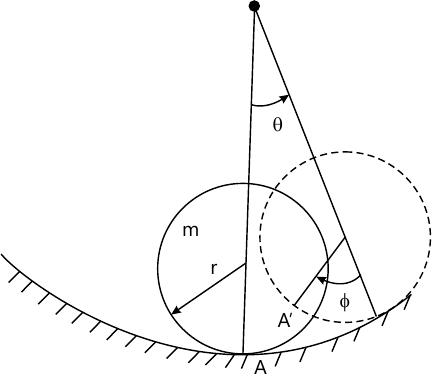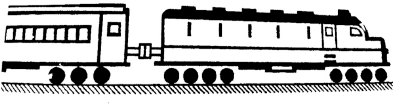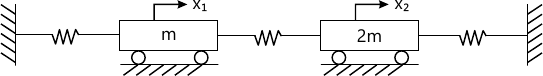MORE IN Dynamics of Machinery
SPPU Mechanical Engineering (Semester 7)
Dynamics of Machinery
December 2015
Total marks: --
Total time: --
INSTRUCTIONS
(1) Assume appropriate data and state your reasons
(2) Marks are given to the right of every question
(3) Draw neat diagrams wherever necessary

Solve any one question from Q1 and Q2
1 (a) The three cylinders of radial engine have a common crank of radius 75mm. The connecting rod length is 225mm. Find the maximum primary and secondary forces when running at 2800rpm if the reciprocating mass per cylinder is 2 kg.
6 M
1 (b) A flywheel of mass 10kg and radius of gyration 0.3 m makes torsional rotations under a torsion spring of stiffness 5Nm/rad. A viscous damper is fitted and it is found that the amplitude is reduced by a factor 100 over any two successive cycles. Find
i) Damping factor
ii) Damping coefficient
iii) Damped frequency
iv) Periodic time of oscillations
4 M

2 (a) Show that primary forces in V-90 engine can be completely balanced.
4 M
2 (b) A cylinder of mass m and radius r rolls without slipping inside a cylinder surface of radius R. Find natural frequency for small oscillations about lowest point.4 M

Solve any one question from Q3 and Q4
3 (a) Explain significance of critical damping. Give any two applications of critical Damping.
4 M
3 (b) Consider the spring-mass-damper system in which mass is given a velocity of 0.1 m/s. What will be the subsequent displacement and velocity of the mass if damping coefficient is 100 Ns/m, spring stiffness is 3000 N/m and mass of 20 kg; F0sin ωt=0.
6 M

4 (a) A body of mass 100kg is suspended on a leaf spring. The system is then vibrate and its natural frequency when measured is 7 rad/s. It is observed that if the initial amplitude is 48 mm, the subsequent amplitudes are 20mm and 32mm. Determine spring stiffness and coulomb damping force.
6 M
4 (b) Plot magnification factor versus frequency ratio curve for different damping conditions and write concluding remarks.
4 M

Solve any one question from Q5 and Q6
5 (a) Explain degenerate system with any two examples.
4 M
5 (b) Fig. shows an engine connected to a compartment by a spring coupling having and effective linear stiffness of 3×106 N/m. The engine weighs 20 tonnes where as the compartment weighs 15 tonnes. Determine the natural frequency of arrangement as shown in Fig.2.12 M

6 (a) Write short note on torsional vibration of geared system.
4 M
6 (b) Determine natural frequency and corresponding mode shapes of given system as shown in Fig.3. Assume each spring stiffness is k.12 M

Solve any one question from Q7 and Q8
7 (a) The static deflection of an automobile on its spring is 10cm under. Find the critical speed when the trailer is travelling over a road with a profile approximated by a sine wave of amplitude 8 cm and wavelength of 16 m. Assume damping to be given by ξ=0.05. What will be the amplitude of vibration at 75 km/hr?
6 M
Write short note on any two of following:
7 (b) (i) Seismic instruments
6 M
7 (b) (ii) FFT analyzer
6 M
7 (b) (iii) Vibration isolations
6 M

8 (a) What are transmissibility curves? Give the significance of these curves.
6 M
8 (b) A commercial type vibration pickup has a natural frequency of 6Hz and a damping factor of 0.65. What is the lowest frequency beyond which the amplitude can be measured within
i) one percent error
ii) two percent error?
6 M
8 (c) The motion of under damped vibratory system (ξ=0.5) is to be recorded by a seismic instrument having natural frequency of 3 r/s. What is the reading of the instruments if the motion is given by the equation: x=2 sin 2t+3 sin 3t.
6 M

Solve any one question from Q9 and Q10
9 (a) Explain the terms
i) Decibel scale
ii) Sound pressure level
iii) Sound power level
iv) Sound intensity level
v) Frequency range of sound sources
10 M
9 (b) Show that as the distance from point source doubles, the sound intensity level decreases by 6 dB.
6 M

10 (a) A worker is exposed to noise according to the following schedule:
Does the daily noise dose is exceeded as per OSHA standards.
 Exposure level [dB] 92 95 97 102 Period of exposure [hrs] 3 2 2 1
6 M
Write short note on any two of following:
10 (b) (i) Noise sources and control in industries
5 M
10 (b) (ii) Mechanism of hearing
5 M
10 (b) (iii Loudness of composite sound
5 M

More question papers from Dynamics of Machinery